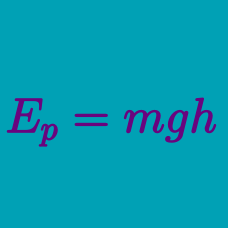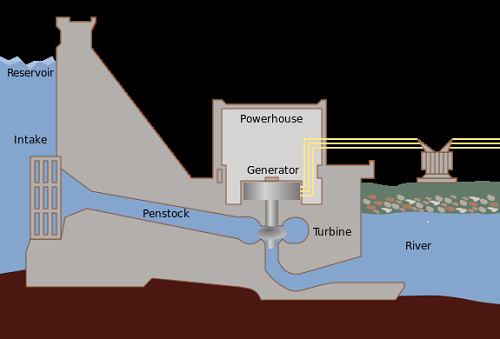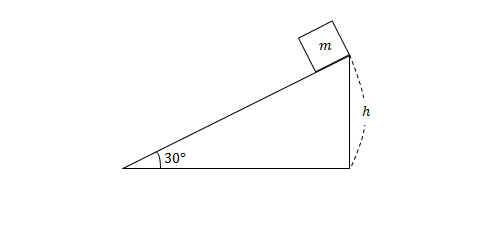Classical Mechanics

Potential energy vs. work

An object of mass $4\text{ kg}$ lies on the ground. If the object is lifted up $20\text{ m},$ what is the work done by gravity?

Assume that gravitational acceleration is $g=10\text{ m/s}^2.$The figure above depicts a dam generator. If the control gate opens, water from the reservoir flows down the penstock and spins the turbine, allowing the generator to produce electricity. Which of the following statements is true concerning this process?A block of mass $m=2\text{ kg}$ initially at rest at the peak of a frictionless inclined plane that makes a $30^\circ$ angle with the horizontal, slides all the way down the ramp. If the initial height of the block was $h=50\text{ m},$ what is the total work done on the block by gravity?

An object of mass $9\text{ kg}$ moves on a horizontal floor at $4\text{ m/s}.$ If the object moves a total of $50\text{ m},$ what is the work done by gravity to the object?

Assume that gravitational acceleration is $g=10\text{ m/s}^2.$

Two identical objects are hanging on each sides of a spring. The spring is stretched by $10\text{ cm}$ and then let go. As the spring shortens back to its original length, which of the following statements is true?

×